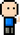# Josh Meets Computer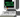# Graph Types

April 2, 2018

Undirected - generally used for symmetric relationships, these graphs have edges between nodes that do not have an orientation (or direction). An example could be a family and friends network — if one person is a family member of another, we can assume that the same is true in the opposite direction.

Directed - these are useful for asymmetric relationships. The edges on these graphs come with a particular direction from one node to the next. These are useful when there is a specific relationship between two nodes, such as an animal ecosystem food network where one animal is the preditor and one is the prey.

Weighted - these have a numerical value assigned to the edge. The weight of an edge can be different for different connections between nodes. This is good if you are trying to count some relationship between two nodes, such as the number of emails sent from one person node to another person node.

Signed - this provides a binary classification of edges between nodes. Each edge has a positive or negative sign, which could denote things like ‘friend’ or ‘enemy’ between two nodes.

Labeled - edges have attributes (labels, colors) to describe the type of edge being used to connect two nodes. For example, describing the nature of the relationship between two nodes in a categorical fashion.

Multi-graph - labeled with multiple edges connecting two nodes. If there are two or more types of relationships between two nodes, there could be multiple edges connecting them. This is useful for Labeled graphs where the relationship between two nodes is defined by more than one category.

``````import math
import networkx as nx
import pandas as pd
import numpy as np
from networkx.algorithms import bipartite

import matplotlib.pyplot as plt
%matplotlib notebook``````

### Graph Plot Helper

``````def plot_graph(G, weight_name=None):
'''
G: a networkx G
weight_name: name of the attribute for plotting edge weights (if G is weighted)
'''
%matplotlib notebook
import matplotlib.pyplot as plt

fig = plt.figure(figsize=(8,8))
fig.suptitle(weight_name)
pos = nx.spring_layout(G)
edges = G.edges()
weights = None
plt.axis('off')

if weight_name:
weights = [int(G[u][v][weight_name]) for u,v in edges]
labels = {i:k for i, k in nx.get_edge_attributes(G, weight_name).items() if k != 0}
nx.draw_networkx_edge_labels(G,pos,edge_labels=labels)
nx.draw_networkx(G, pos, edges=edges, width=weights, alpha=0.7);
else:
nx.draw_networkx(G, pos, edges=edges, alpha=0.7);``````
``help(relG.add_edge)``
``````Help on method add_edge in module networkx.classes.graph:

add_edge(u, v, attr_dict=None, **attr) method of networkx.classes.graph.Graph instance
Add an edge between u and v.

The nodes u and v will be automatically added if they are

Edge attributes can be specified with keywords or by providing
a dictionary with key/value pairs.  See examples below.

Parameters
----------
u, v : nodes
Nodes can be, for example, strings or numbers.
Nodes must be hashable (and not None) Python objects.
attr_dict : dictionary, optional (default= no attributes)
Dictionary of edge attributes.  Key/value pairs will
update existing data associated with the edge.
attr : keyword arguments, optional
Edge data (or labels or objects) can be assigned using
keyword arguments.

--------

Notes
-----

Many NetworkX algorithms designed for weighted graphs use as
the edge weight a numerical value assigned to a keyword
which by default is 'weight'.

Examples
--------
The following all add the edge e=(1,2) to graph G:

>>> G = nx.Graph()   # or DiGraph, MultiGraph, MultiDiGraph, etc
>>> e = (1,2)
>>> G.add_edge(1, 2)           # explicit two-node form
>>> G.add_edge(*e)             # single edge as tuple of two nodes

Associate data to edges using keywords:

>>> G.add_edge(1, 3, weight=7, capacity=15, length=342.7)``````

## Employee Relationships and Shared Movie Interests

The challenge set in front of me is to determine if there is a correlation between employees movie interests and their relationship with one another. I have data that measures employee relationships, and I have data that recorded the movies each employee has watched. The plan is to correlate the employee relationship attributes with the shared movie interest attributes.

``````# Load in employees and movies as graph -- edges connect movies seen by employees
emp_movG = nx.from_pandas_dataframe(emp_movDF, *emp_movDF.columns)

# Look at all the different graph layout styles
pos_options = [x for x in nx.__dir__() if x.endswith('_layout')]
plot_count = len(pos_options)
fig = plt.figure(figsize=(plot_count,plot_count*4))

for i, p in enumerate(pos_options):
pos = getattr(nx, p)(emp_movG)
ax.set_title(p)
ax.get_xaxis().set_visible(False)
ax.get_yaxis().set_visible(False)
nx.draw_networkx(emp_movG, pos, ax=ax)

plt.tight_layout()
plt.show()``````
``<IPython.core.display.Javascript object>``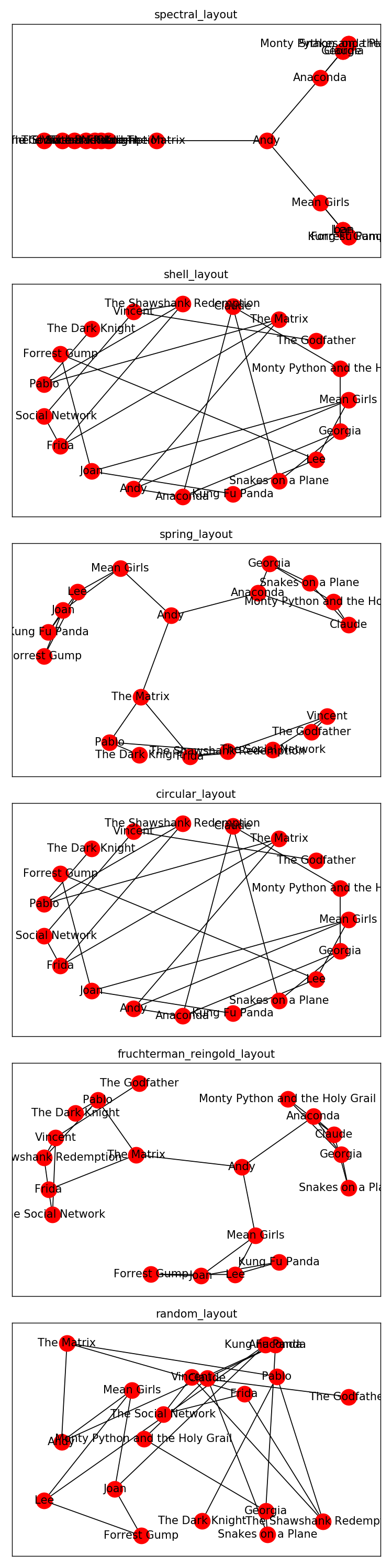``````G = nx.Graph()

plot_graph(G, weight_name='weight')``````
``<IPython.core.display.Javascript object>``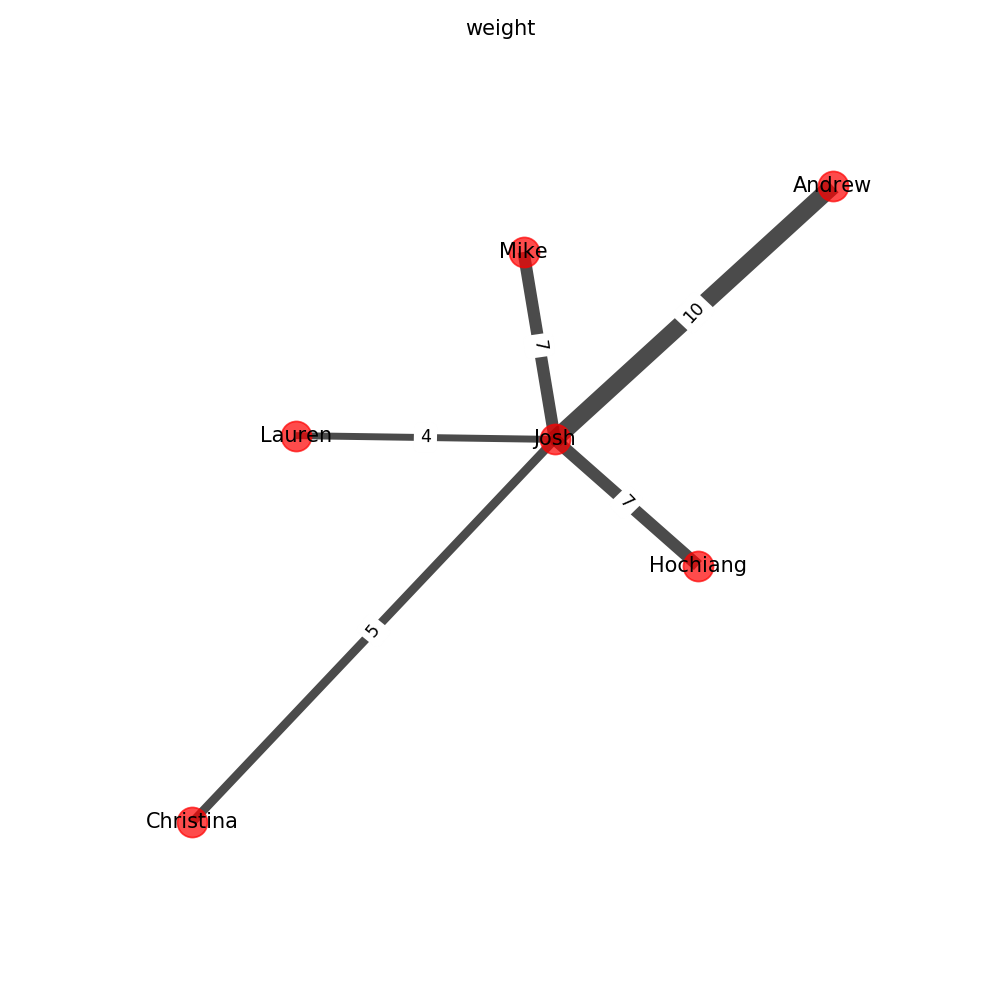``````# Get left and right bipartite nodes
mov_nodes = bipartite.sets(emp_movG)
emp_nodes = bipartite.sets(emp_movG)

print('List of Movies','\n---------------')
for i in mov_nodes: print(i)
print()
print('List of Employees','\n----------------')
for i in emp_nodes: print(i)

print()
print('Employee Movie Choices file','\n----------------------')
!cat Employee_Movie_Choices.txt
print()
print('Employee Relationship file','\n----------------------')
!cat Employee_Relationships.txt``````
``````List of Movies
---------------
Mean Girls
The Dark Knight
Monty Python and the Holy Grail
The Matrix
The Shawshank Redemption
The Godfather
Forrest Gump
The Social Network
Anaconda
Kung Fu Panda
Snakes on a Plane

List of Employees
----------------
Joan
Claude
Lee
Vincent
Pablo
Frida
Georgia
Andy

Employee Movie Choices file
----------------------
#Employee	Movie
Andy	Anaconda
Andy	Mean Girls
Andy	The Matrix
Claude	Anaconda
Claude	Monty Python and the Holy Grail
Claude	Snakes on a Plane
Frida	The Matrix
Frida	The Shawshank Redemption
Frida	The Social Network
Georgia	Anaconda
Georgia	Monty Python and the Holy Grail
Georgia	Snakes on a Plane
Joan	Forrest Gump
Joan	Kung Fu Panda
Joan	Mean Girls
Lee	Forrest Gump
Lee	Kung Fu Panda
Lee	Mean Girls
Pablo	The Dark Knight
Pablo	The Matrix
Pablo	The Shawshank Redemption
Vincent	The Godfather
Vincent	The Shawshank Redemption
Vincent	The Social Network

Employee Relationship file
----------------------
Andy	Claude	0
Andy	Frida	20
Andy	Georgia	-10
Andy	Joan	30
Andy	Lee	-10
Andy	Pablo	-10
Andy	Vincent	20
Claude	Frida	0
Claude	Georgia	90
Claude	Joan	0
Claude	Lee	0
Claude	Pablo	10
Claude	Vincent	0
Frida	Georgia	0
Frida	Joan	0
Frida	Lee	0
Frida	Pablo	50
Frida	Vincent	60
Georgia	Joan	0
Georgia	Lee	10
Georgia	Pablo	0
Georgia	Vincent	0
Joan	Lee	70
Joan	Pablo	0
Joan	Vincent	10
Lee	Pablo	0
Lee	Vincent	0
Pablo	Vincent	-20``````
``````# Assign node attributes (employees and movies)
nx.set_node_attributes(emp_movG, 'type', {i:'movie' for i in mov_nodes})
nx.set_node_attributes(emp_movG, 'type', {i:'employee' for i in emp_nodes})``````

### Projected Graph of Shared Movie Interests

``````# Get Projected graph of employees, seeing what movie similaries there are
emp_projected = bipartite.weighted_projected_graph(emp_movG, emp_nodes)
plot_graph(emp_projected, 'weight')``````
``<IPython.core.display.Javascript object>``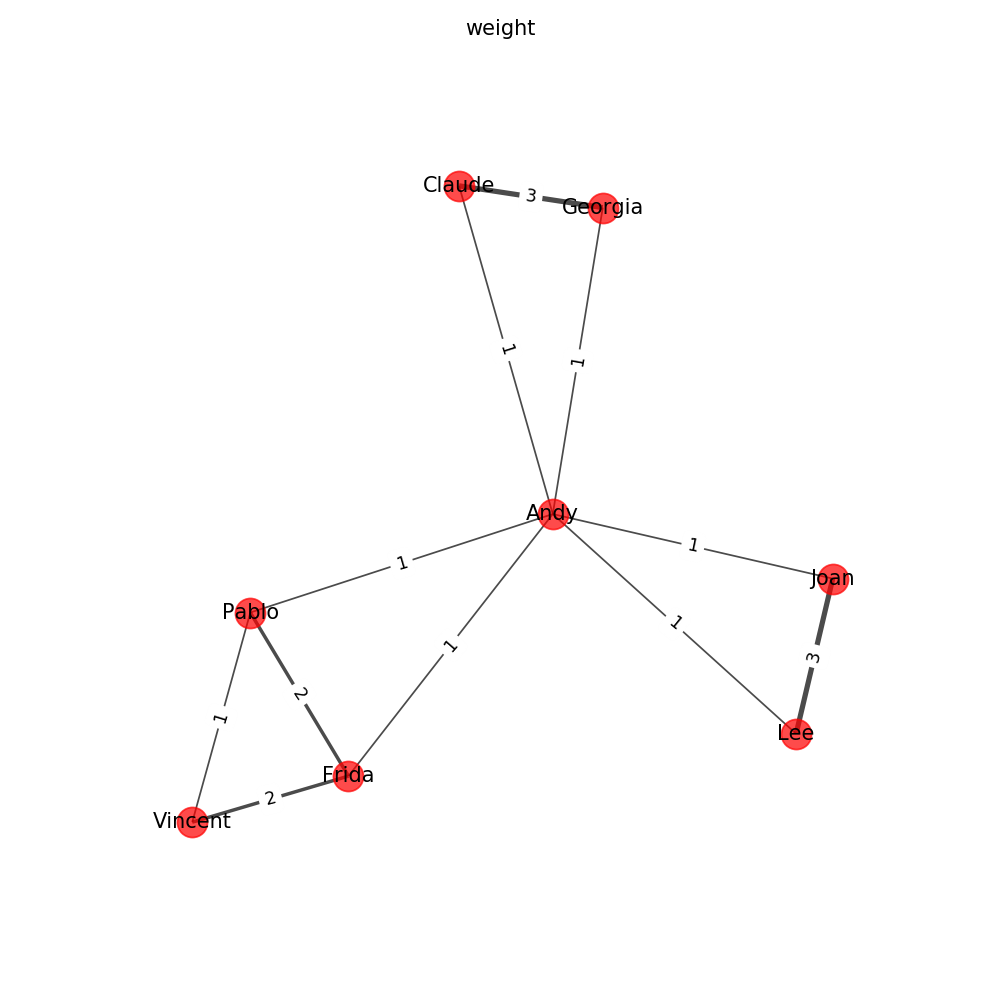``````# Load in regular graph - Relationships
relDF = pd.read_csv('Employee_Relationships.txt', sep='\t', names=['e1', 'e2', 'relationship'])
relG = nx.from_pandas_dataframe(relDF, *relDF.columns)
max_weight = max(relDF['relationship'])

plt.figure('Weighted Relationships between coworkers', figsize=(8,8))
pos = nx.spring_layout(relG)
edges = relG.edges()
plt.axis('off')

friends = ['green' if x['relationship'] > 0 else 'red' for x in relG.edges(data=True)]
weights = [int(relG[u][v]['relationship'])**2/max_weight**2 * 10 for u,v in edges]
labels = nx.get_edge_attributes(relG, 'relationship')
# nx.draw_networkx_edge_labels(relG,pos, edge_labels=labels)
nx.draw_networkx(relG, pos, edges=edges, width=weights, alpha=0.7, edge_color=friends, node_color=node_color);
plt.show()``````
``<IPython.core.display.Javascript object>``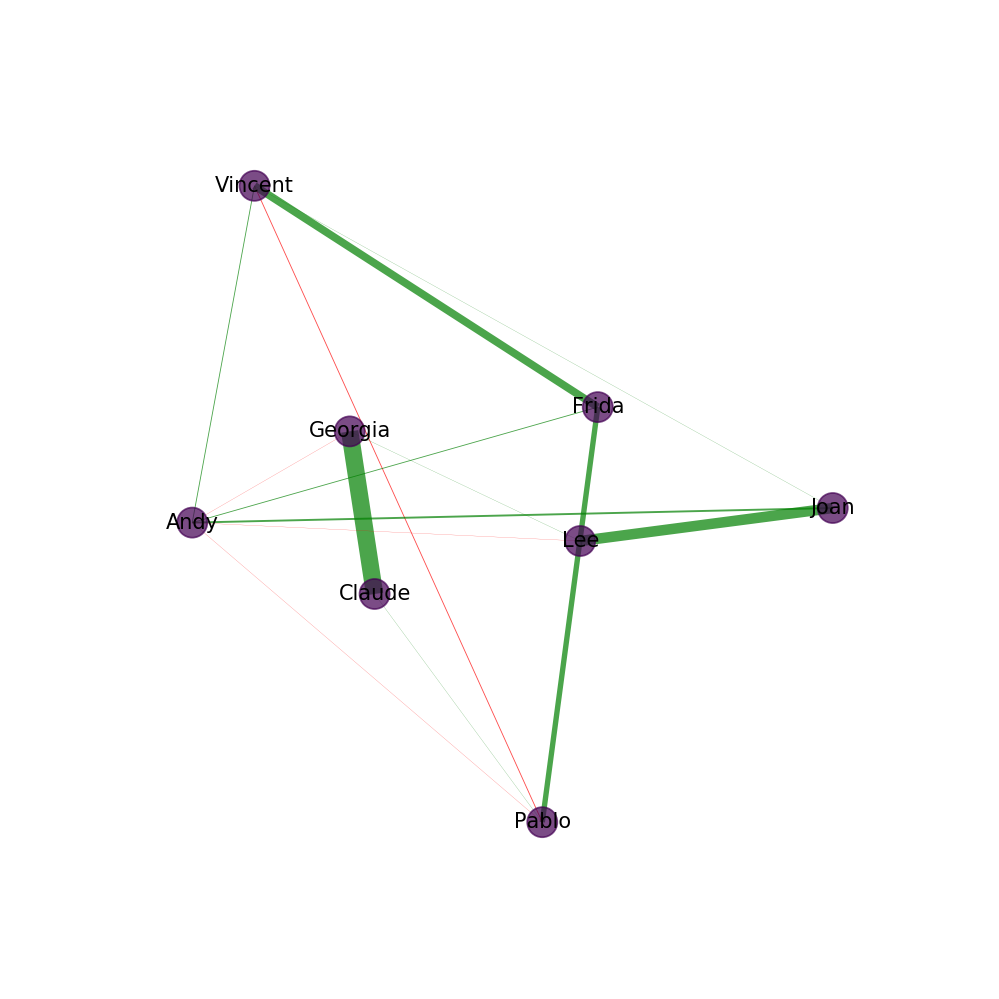### Add Shared Movie Interest to Relationship Graph

``````# Add shared movie interest attributes from projected graph
weight_attr = {(i, i): i for i in emp_projected.edges(data='weight')}
nx.set_edge_attributes(relG, 'shared_movies', 0)
nx.set_edge_attributes(relG, 'shared_movies', weight_attr)

plot_graph(relG, 'shared_movies')``````
``<IPython.core.display.Javascript object>``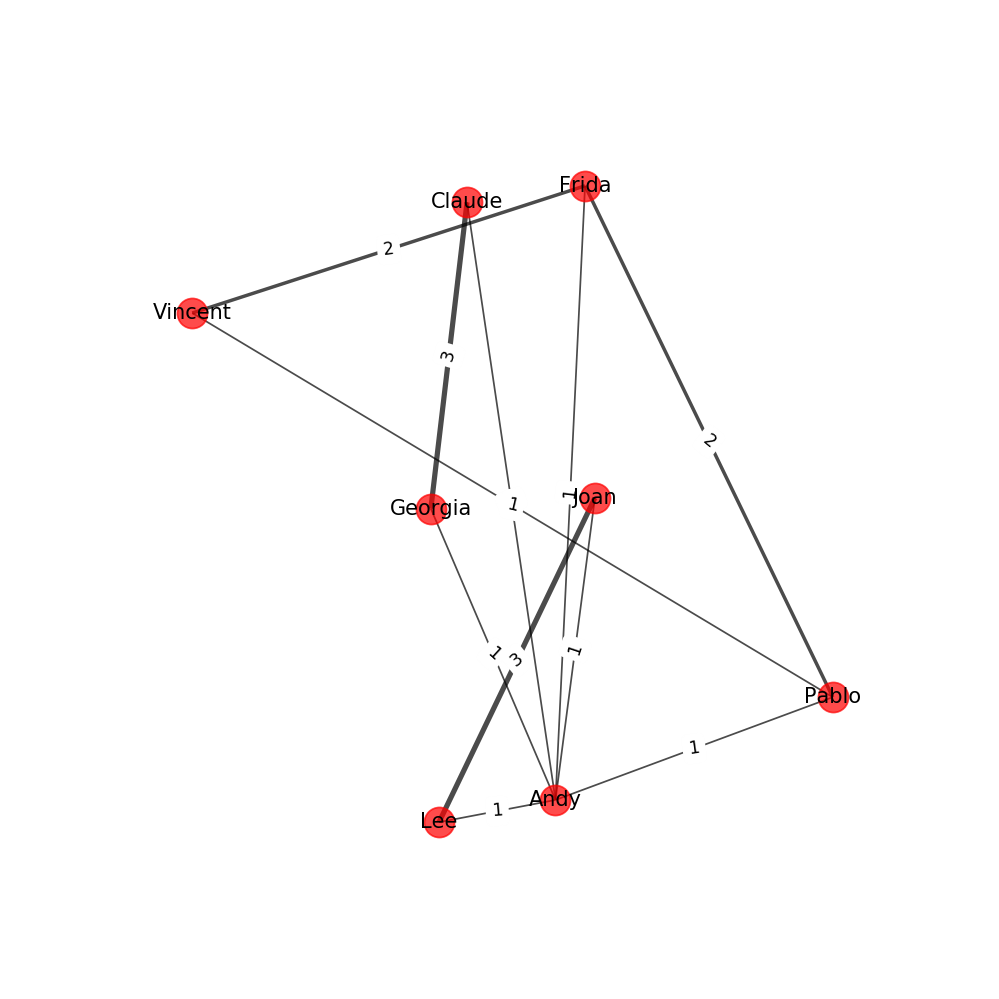### Convert Attributes (Movie, Relationship) To DataFrame and correlate

``````# Create two column DF on the attributes of graph edges
idx = [(i, i) for i in relG.edges(data=True)]
rel = [i for i in relG.edges(data='relationship')]
mov = [i for i in relG.edges(data='shared_movies')]
corrDF = pd.DataFrame({'relationship':rel, 'shared_movies':mov}, index=idx)

print('Final Correlation Between relationships and movie interests:')
# Determine correlation between relationships and shared movies
corrDF.corr('pearson')``````
``Final Correlation Between relationships and movie interests:``
relationship shared_movies
relationship 1.000000 0.788396
shared_movies 0.788396 1.000000

# Further Explanation of Graphs Used

## Bipartite Graphs

Unique Graph structure where nodes can be split into a left node subgroup and a right node subgroup, where the left nodes are connected only to the right nodes and visa versa. For example, the defensive positions on a football team may be connected to a particular offensive position, but no defensive position is assigned to guard another defensive position.

``from networkx.algorithms import bipartite``
``````B = nx.Graph()
B.add_edges_from([('A',1), ('B',2), ('C',3), ('D',4), ('D', 2), ('A', 3)])

bipartite.is_bipartite(B)``````
``True``
``bipartite.sets(B)``
``({1, 2, 3, 4}, {'A', 'B', 'C', 'D'})``

## Projected Graphs

Within the subgroups of Bipartitite Graphs, there can be Projected Graphs describing the relationship amongst the left and right Bipartite subgroups. For example, in a Bipartite Graph describing fans and their favorite sports teams (left and right Bipartite), you could create a Projected Graph on the fans describing which fans support the same teams, where the edges between fans desribes the number or category of team two fan nodes have in common. These kind of relationships can be useful for viral marketing, finding similarities amongst individuals and customizing marketing material accordingly.

``````X1 = set(['A', 'B', 'C', 'D'])
P = bipartite.weighted_projected_graph(B, X1)
print('Described share connections amongst L-group in Bipartite Graph')
P.edges(data=True)``````
``````Described share connections amongst L-group in Bipartite Graph

[('B', 'D', {'weight': 1}), ('C', 'A', {'weight': 1})]``````